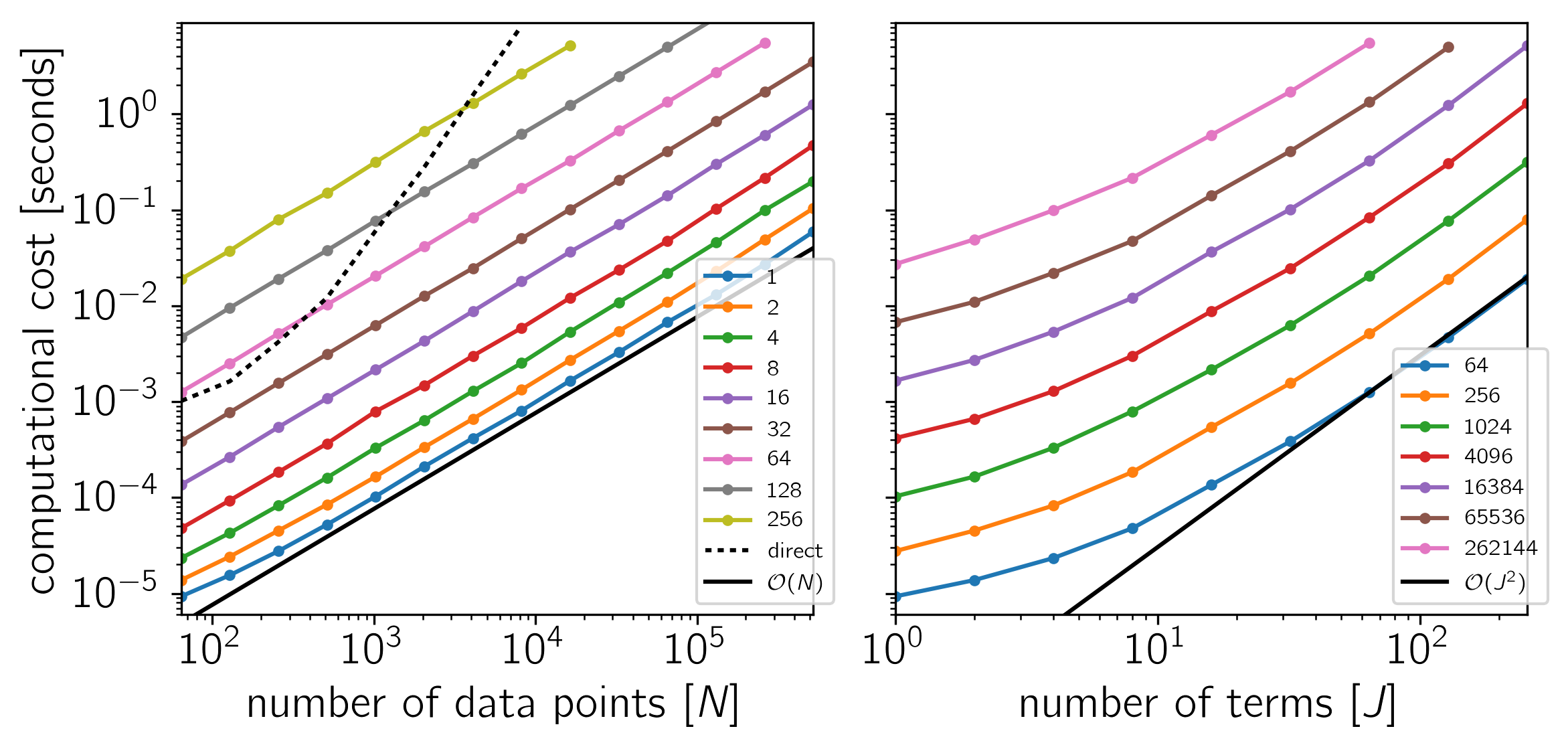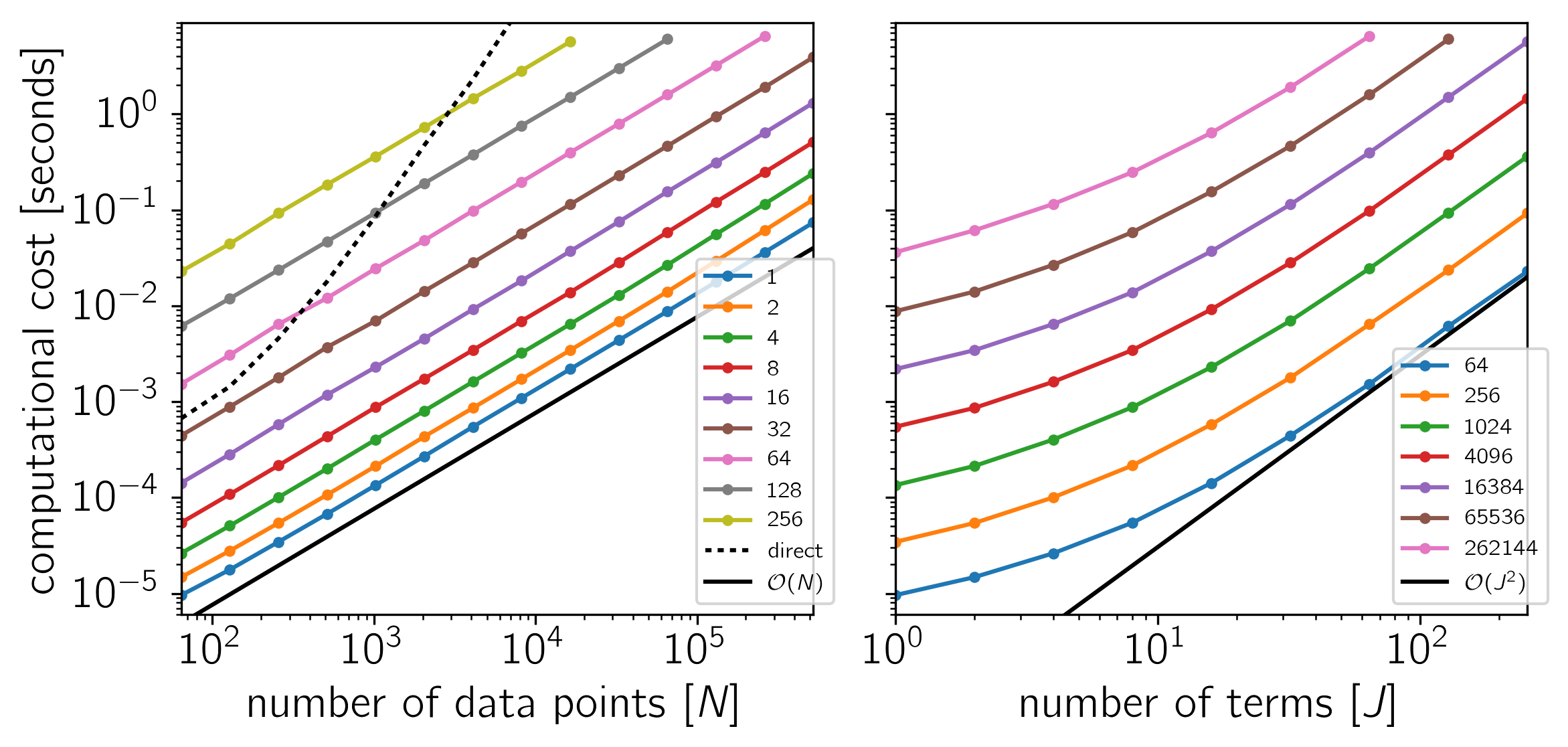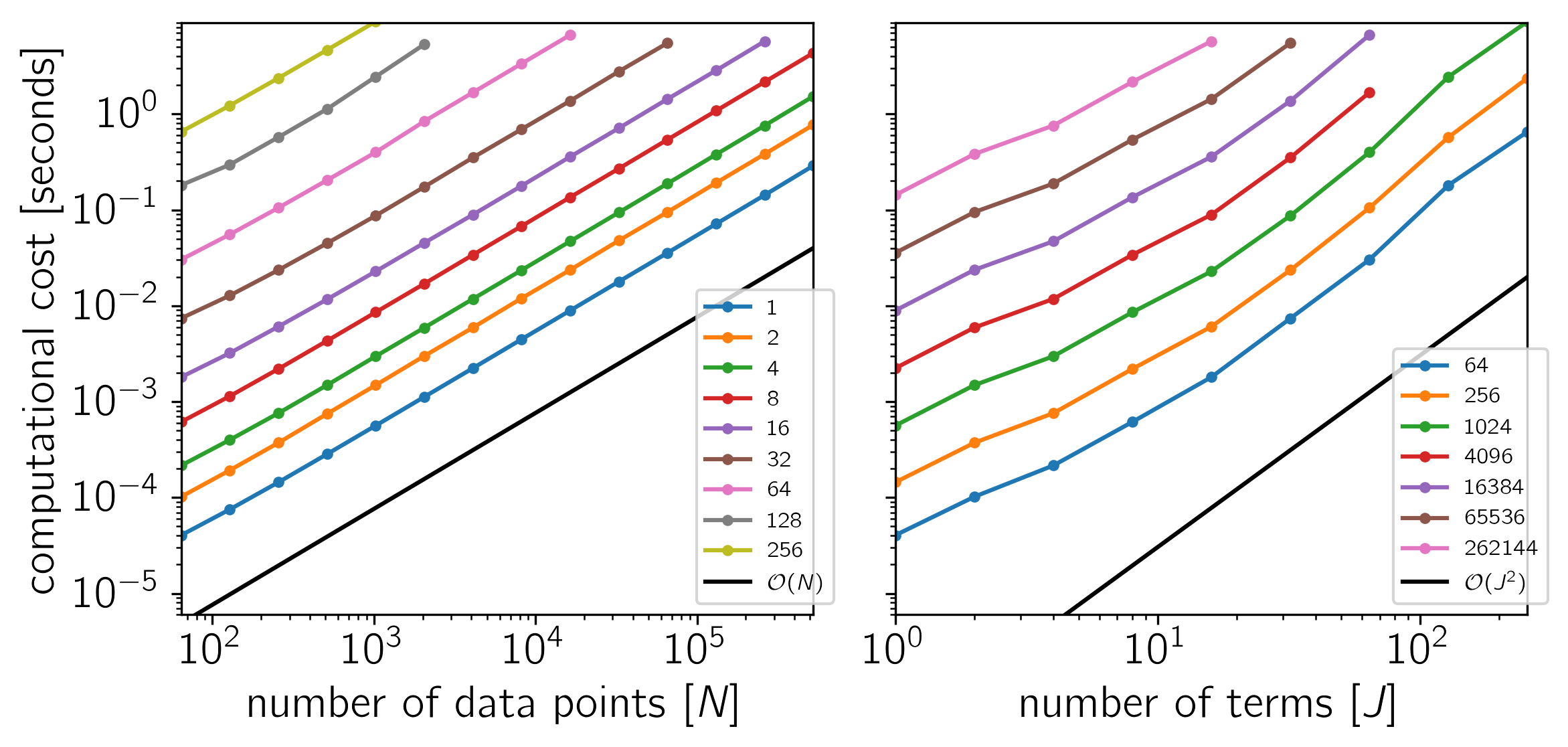# Benchmark¶

To test the scaling of the celerite algorithm, the following figures show the computational cost of

gp.compute(x, yerr)
gp.log_likelihood(y)


as a function of the number of data points $$N$$ and the number of terms $$J$$. The theoretical scaling of this algorithm should be $$\mathcal{O}(N\,J^2)$$.

The code for this example is available on GitHub and below we show the results for a few different systems. In each plot, the computational time is plotted as a function of $$N$$ or $$J$$ and the colors correspond to the other dimension as indicated by the legend.

Example 1: On macOS with Eigen 3.3.3, pybind11 2.0.1, and NumPy 1.12.0.Example 2: On Linux with Eigen 3.3.3, pybind11 2.0.1, and NumPy 1.12.0.Example 3: For comparison, a CARMA solver using a Kalman filter. On Linux with Eigen 3.3.3, pybind11 2.0.1, and NumPy 1.12.0.